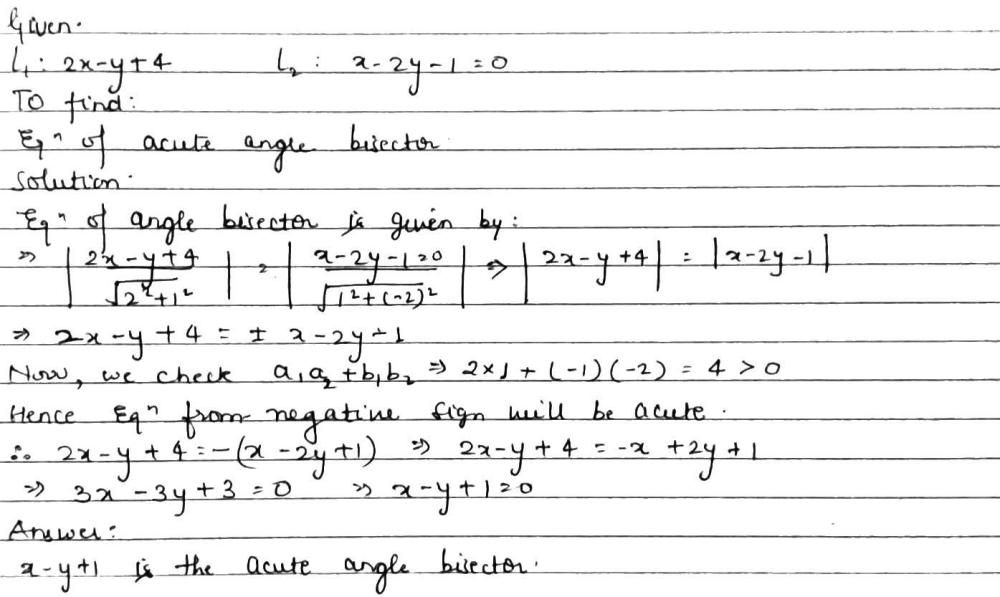Home/Class 11/Maths/

## QuestionMathsClass 11

The equation of the bisector of the acute angle between the lines $$2x-y+4=0$$and
$$x-2y=1$$is
(a) $$x-y+5=0$$ (b)$$x-y+1=0$$ (c)$$x-y=5$$ (d) none of these